Get SGD58.85 off your premium account! Valid till 9 August 2021. Use the Code ‘SGLEARN2021’ upon checkout. Click Here

## From-scratch implementation of AlphaZero for Connect4

Step-by-step illustration on how one can implement AlphaZero on games using just PyTorch and standard python libraries

In 2016, Google DeepMind created a big stir when its computer program AlphaGo defeated reigning Go world champion Lee Sedol 4–1 in a match watched by millions, a feat never before achieved by any computer program in the ultra-complicated game of Go which has been dominated by humans until then. However, AlphaGo Zero, published by DeepMind about a year later in 2017, pushed boundaries one big step further by achieving a similar feat without any human data inputs. (AlphaGo referenced Go Grandmaster games for initial training) A subsequent paper released by the same group successfully applied the same reinforcement learning + supervised learning framework to chess, outperforming the previous best chess program Stockfish after just 4 hours of training.

Awed by the power of such reinforcement learning models, I wanted to understand how it works to gain some insights, and there’s nothing better than trying to build my own chess AI program from scratch, closely following the methods as described in the papers above. However, things quickly got too expensive to bear, as even though the program was up and running, training it to a reasonable skill level would most likely require millions in terms of GPU and TPU costs.

Unable to match the deep pockets of Google, I decided to try to implement AlphaZero on Connect4 instead, a game which is much simpler than chess and would be more gentle on computational power. The point here, is to demonstrate that the AlphaZero algorithm works well to create a powerful Connect4 AI program, eventually. The implementation scripts on the methods described here are all available on my Github repo.

### The Connect4 Board

Firstly, we need to create the Connect4 board in Python for us to play around with. I’ve created a class called “board” with 4 methods ”__init__”, “drop_piece”, “check_winner”, “actions”.

class board():
def __init__(self):
self.init_board = np.zeros([6,7]).astype(str)
self.init_board[self.init_board == "0.0"] = " "
self.player = 0
self.current_board = self.init_board

1) “__init__” constructor initializes an empty Connect4 board of 6 rows and 7 columns as an np.array, stores the board state as well as the current player to play

2) “drop_piece” updates the board with “X” or “O” as each player plays

3) “check_winner” returns True if somebody wins in the current board state

4) “actions” returns all possible moves which can be played given the current board state, so that no illegal moves are played

### The Big Picture

There are 3 key components in AlphaZero, and I will describe their implementations in more detail later. They are:

1) Deep convolutional residual neural network
Input : Connect 4 board state
Outputs : policy(probability distribution of possible moves), value(O wins: +1, X wins:-1, draw:0)

2) Monte-Carlo Tree Search (MCTS)
Self-play guided by policy from neural network to generate games dataset to train neural network, in an iterative process

3) Evaluate neural network
﻿Player vs player, each guided by current net and previous net respectively, retain net that wins the match for next iteration

### Deep Convolutional Residual Neural Network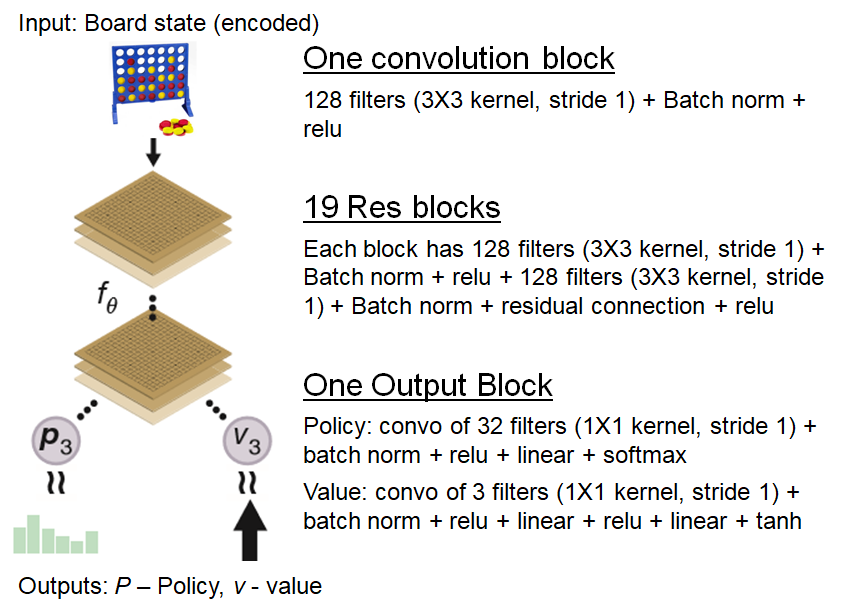Neural network architecture of AlphaZero used here

We use a deep convolutional residual neural network (using PyTorch) with the above architecture similar to AlphaZero to map an input Connect4 board state to its associated policy and value. The policy is essentially a probability distribution of next moves the player should move from the current board state (the strategy), and the value represents the probability of the current player winning from that board state. This neural net is an integral part of the MCTS, where it helps guide the tree search via its policy and value outputs as we will see later. We build this neural net (which I call ConnectNet) using one initial convolution block, followed by 19 residual blocks and finally one output block as detailed below.

Convolutional Block

class ConvBlock(nn.Module):
def __init__(self):
super(ConvBlock, self).__init__()
self.action_size = 7
self.conv1 = nn.Conv2d(3, 128, 3, stride=1, padding=1)
self.bn1 = nn.BatchNorm2d(128)

def forward(self, s):
s = s.view(-1, 3, 6, 7)  # batch_size x channels x board_x x board_y
s = F.relu(self.bn1(self.conv1(s)))
return s

Residual Block

class ResBlock(nn.Module):
def __init__(self, inplanes=128, planes=128, stride=1, downsample=None):
super(ResBlock, self).__init__()
self.conv1 = nn.Conv2d(inplanes, planes, kernel_size=3, stride=stride,
self.bn1 = nn.BatchNorm2d(planes)
self.conv2 = nn.Conv2d(planes, planes, kernel_size=3, stride=stride,
self.bn2 = nn.BatchNorm2d(planes)

def forward(self, x):
residual = x
out = F.relu(self.bn1(self.conv1(x)))
out = self.bn2(self.conv2(out))
out += residual
out = F.relu(out)
return out

Output Block

class OutBlock(nn.Module):
def __init__(self):
super(OutBlock, self).__init__()
self.conv = nn.Conv2d(128, 3, kernel_size=1) # value head
self.bn = nn.BatchNorm2d(3)
self.fc1 = nn.Linear(3*6*7, 32)
self.fc2 = nn.Linear(32, 1)

self.conv1 = nn.Conv2d(128, 32, kernel_size=1) # policy head
self.bn1 = nn.BatchNorm2d(32)
self.logsoftmax = nn.LogSoftmax(dim=1)
self.fc = nn.Linear(6*7*32, 7)

def forward(self,s):
v = F.relu(self.bn(self.conv(s))) # value head
v = v.view(-1, 3*6*7)  # batch_size X channel X height X width
v = F.relu(self.fc1(v))
v = torch.tanh(self.fc2(v))

p = F.relu(self.bn1(self.conv1(s))) # policy head
p = p.view(-1, 6*7*32)
p = self.fc(p)
p = self.logsoftmax(p).exp()
return p, v

Putting it altogether

class ConnectNet(nn.Module):
def __init__(self):
super(ConnectNet, self).__init__()
self.conv = ConvBlock()
for block in range(19):
setattr(self, "res_%i" % block,ResBlock())
self.outblock = OutBlock()

def forward(self,s):
s = self.conv(s)
for block in range(19):
s = getattr(self, "res_%i" % block)(s)
s = self.outblock(s)
return s

The raw Connect4 board is encoded into a 6 by 7 by 3 matrix of 1’s and 0’s before input into the neural net, where the 3 channels each of board dimensions 6 by 7 encode the presence of “X”, “O” (1 being present and 0 being empty), and player to move (0 being “O” and 1 being “X”), respectively.

### Encoder to encode Connect4 board for neural net input
def encode_board(board):
board_state = board.current_board
encoded = np.zeros([6,7,3]).astype(int)
encoder_dict = {"O":0, "X":1}
for row in range(6):
for col in range(7):
if board_state[row,col] != " ":
encoded[row, col, encoder_dict[board_state[row,col]]] = 1
if board.player == 1:
encoded[:,:,2] = 1 # player to move
return encoded

Finally, to properly train this neural net which has a two-headed output, a custom loss function (AlphaLoss) is defined as simply the sum of the mean-squared error value and cross-entropy policy losses.

### Neural Net loss function implemented via PyTorch
class AlphaLoss(torch.nn.Module):
def __init__(self):
super(AlphaLoss, self).__init__()

def forward(self, y_value, value, y_policy, policy):
value_error = (value - y_value) ** 2
policy_error = torch.sum((-policy*
(1e-8 + y_policy.float()).float().log()), 1)
total_error = (value_error.view(-1).float() + policy_error).mean()
return total_error

### Monte-Carlo Tree Search

A game can be described as a tree in which the root is the board state and its branches are all the possible states that can result from it. In a game such as Go where the number of branches increase exponentially as the game progresses, it is practically impossible to simply brute-force evaluate all branches. Hence, the Monte-Carlo Tree Search (MCTS) algorithm is devised to search in a smarter and more efficient way. Essentially, one wants to optimize the exploration-exploitation tradeoff, where one wants to search just exhaustively enough (exploration) to discover the best possible reward (exploitation). This is succinctly described in a single equation in the MCTS algorithm that defines the upper confidence bound (UCB):

Here, Q is the mean action value (average reward), cpuct is a constant determining the level of exploration (set as 1), P(s=state,a=action) is the prior probability of choosing action given by the policy output of the neural net, N(s,a) is the number of times the branch corresponding to action has been visited. The N sum over b in the numerator sums over all explored branches (actions) from state s which is essentially the number of times the parent of (s,a) has been visited.

The MCTS algorithm proceeds in the following steps.

1. Select
### Recursively selects the nodes based on highest UCB (best move) until leaf node or terminal node is reached. Adds ###node of best move if its not yet created.
def select_leaf(self):
current = self
while current.is_expanded:
best_move = current.best_child()
return current

Starting from s, the search selects the next branch that has the highest UCB, until a leaf node ( a state in which none of its branches have yet been explored) or a terminal node (end game state) is reached. We can see that if the reward Q is high, then it is more likely to choose that branch. The second exploration term also plays a big part, where we see that if action is only visited a few times, then this term would be large and the algorithm is then more likely to choose the associated branch. The neural net guides the selection by providing the prior probability P, which initially would be random when the neural network is untrained.

2. Expand and Evaluate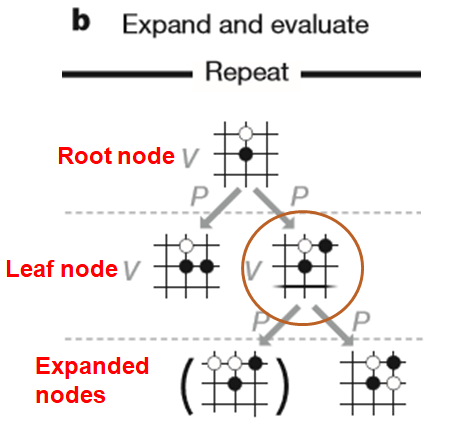Expand and Evaluate — AlphaGo Zero

### Expand only nodes that result from legal moves, mask illegal moves and add Dirichlet noise to prior probabilities of ###root node.
def expand(self, child_priors):
self.is_expanded = True
action_idxs = self.game.actions(); c_p = child_priors
if action_idxs == []:
self.is_expanded = False
self.action_idxes = action_idxs
# mask all illegal actions
for i in range(len(child_priors)):
if i not in action_idxs:
c_p[i] = 0.0000000000
# add dirichlet noise to child_priors in root node
if self.parent.parent == None:
self.child_priors = c_p

Here, the leaf node is expanded by evaluating the states associated with the expanded nodes with the neural net to obtain and store P. Of course, illegal moves should not be expanded and will be masked (by setting prior probabilities to zero). We will also add Dirichlet noise here if the node is a root node to provide randomness to the exploration so that every MCTS simulation would be likely different.

3. Backup

### Recursively update the visits counts and values of nodes once leaf node is evaluated.
def backup(self, value_estimate: float):
current = self
while current.parent is not None:
current.number_visits += 1
if current.game.player == 1: # same as current.parent.game.player = 0
current.total_value += (1*value_estimate) # value estimate +1 = O wins
elif current.game.player == 0: # same as current.parent.game.player = 1
current.total_value += (-1*value_estimate)
current = current.parent

Now, the leaf node is evaluated by the neural net to determine its value v. This value v is then used to update the average v of all parent nodes above it. The update should be such that O and X would play to their best (Minimax) eg. If O wins (v = +1 evaluated for leaf node), then in the direct parent node of this leaf node it would be O’s turn to play and we would update v = +1 for this parent node, then update v = -1 for all other parent nodes where X is to play to denote that this action is bad for X. Finally, update v = 0 in case of a draw.

### Code snippet for each simulation of Select, Expand and Evaluate, and Backup. num_reads here is the parameter ###controlling the number of simulations.
root = UCTNode(game_state, move=None, parent=DummyNode())
for i in range(num_reads):
leaf = root.select_leaf()
encoded_s = ed.encode_board(leaf.game); encoded_s = encoded_s.transpose(2,0,1)
encoded_s = torch.from_numpy(encoded_s).float().cuda()
child_priors, value_estimate = net(encoded_s)
child_priors = child_priors.detach().cpu().numpy().reshape(-1); value_estimate = value_estimate.item()
if leaf.game.check_winner() == True or leaf.game.actions() == []: # if somebody won or draw
leaf.backup(value_estimate); continue
leaf.expand(child_priors) # need to make sure valid moves
leaf.backup(value_estimate)
return root

The above process of Select, Expand and Evaluate and Backup represents one search path or simulation for each root node for the MCTS algorithm. In AlphaGo Zero, 1600 such simulations are done. For our Connect4 implementation, we only run 777 since it’s a much simpler game. After running 777 simulations for that root node, we will then formulate the policy p for the root node which is defined to be proportional to the number of visits of its direct child nodes. This policy p will then be used to select the next move to the next board state, and this board state will then be treated as the root node for next MCTS simulations and so on until the game terminates when someone wins or draw. The whole procedure in which one runs MCTS simulations for each root node as one moves through until the end of the game is termed as MCTS self-play.

### Function to execute MCTS self-play
def MCTS_self_play(connectnet,num_games,cpu):
for idxx in range(0,num_games):
current_board = c_board()
checkmate = False
dataset = [] # to store state, policy, value for neural network training
states = []; value = 0; move_count = 0
# play game against self
while checkmate == False and current_board.actions() != []:
# set temperature parameter
if move_count < 11:
t = 1
else:
t = 0.1
states.append(copy.deepcopy(current_board.current_board))
board_state = copy.deepcopy(ed.encode_board(current_board))
root = UCT_search(current_board,777,connectnet,t) # run 777 MCTS simulations
policy = get_policy(root, t); print(policy) # formulate policy based on results of MCTS simulations
current_board = do_decode_n_move_pieces(current_board,\
np.random.choice(np.array([0,1,2,3,4,5,6]), \
p = policy)) # decode action and make a move
dataset.append([board_state,policy]) # stores s, p
print(current_board.current_board,current_board.player); print(" ")
if current_board.check_winner() == True: # if somebody won, update v
if current_board.player == 0: # X wins
value = -1
elif current_board.player == 1: # O wins
value = 1
checkmate = True
move_count += 1
dataset_p = []
# update v for all (s, p) except for starting board state s
for idx,data in enumerate(dataset):
s,p = data
if idx == 0:
dataset_p.append([s,p,0])
else:
dataset_p.append([s,p,value])
del dataset
# save (s,p,v) datasets for neural net training
save_as_pickle("dataset_cpu%i_%i_%s" % (cpu,idxx, datetime.datetime.today().strftime("%Y-%m-%d")),dataset_p)


In each step of the MCTS self-play where a MCTS simulation is run, we will have a board state s, its associated policy p, and value v, hence when the MCTS self-play game finishes, one will have a set of (s, p, v) values. These set of (s, p, v) values will then be used to train the neural network to improve its policy and value prediction, and this trained neural network will then be used to guide the subsequent MCTS iteratively. In this way, one can see that eventually after many, many iterations, the neural net and MCTS together would be very good at generating optimal moves.

### Evaluate Neural Network

After one iteration in which the neural net is trained using MCTS self-play data, this trained neural net is then pitted against its previous version, again using MCTS guided by the respective neural net. The neural network that performs better (eg. Wins the majority of games) would then be used for the next iteration. This ensures that the net is always improving.

### Iteration Pipeline

In summary, a full iteration pipeline consists of:

1. Self-play using MCTS to generate game datasets (spv), with the neural net guiding the search by providing the prior probabilities in choosing the action

2. Train the neural network using the (spv) datasets generated from MCTS self-play

3. Evaluate the trained neural net (at predefined epoch checkpoints) by pitting it against the neural net from the previous iteration, again using MCTS guided by the respective neural nets, and keep only the neural net that performs better.

4. Rinse and repeat

### Results

Iteration 0:
alpha_net_0 (Initialized with random weights)
151 games of MCTS self-play generated

Iteration 1:
alpha_net_1 (trained from iteration 0)
148 games of MCTS self-play generated

Iteration 2:
alpha_net_2 (trained from iteration 1)
310 games of MCTS self-play generated

Evaluation 1:
Alpha_net_2 is pitted against alpha_net_0
Out of 100 games played, alpha_net_2 won 83 and lost 17

Iteration 3:
alpha_net_3 (trained from iteration 2)
584 games of MCTS self-play generated

Iteration 4:
alpha_net_4 (trained from iteration 3)
753 games of MCTS self-play generated

Iteration 5:
alpha_net_5 (trained from iteration 4)
1286 games of MCTS self-play generated

Iteration 6:
alpha_net_6 (trained from iteration 5)
1670 games of MCTS self-play generated

Evaluation 2:
Alpha_net_6 pitted against alpha_net_3
Out of 100 games played, alpha_net_6 won 92 and lost 8.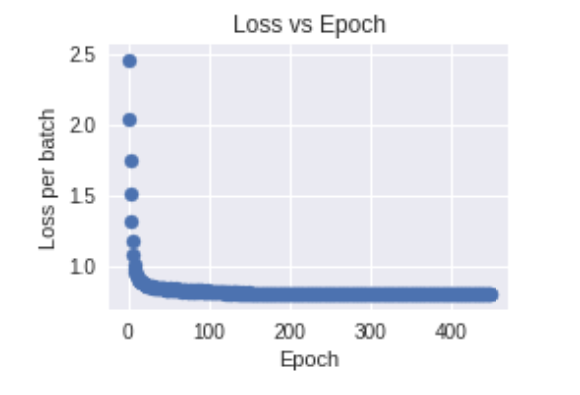Typical Loss vs Epoch for neural net training at each iteration.

Over a period of several weeks of sporadic training on Google Colab, a total of 6 iterations for a total of 4902 MCTS self-play games was generated. A typical loss vs epoch of the neural network training for each iteration is shown above, showing that training proceeds quite well. From both evaluations 1 & 2 at selected points in the iterations, we can see that the neural net is indeed always improving and becoming stronger than its previous version in generating winning moves.

Now is probably time to show some actual games! The gif below shows an example game between alpha_net_6 (playing as X) and alpha_net_3 (playing as O), where X won.

At this moment, I am still training net/running MCTS self-play. I hope to be able to reach a stage whereby the MCTS + net are able to generate perfect moves (Connect4 is a solved game, such that the player that moves first can always force a win), but who knows how many iterations that would need…

Anyway, that’s all folks! Hope that you would find this post interesting and useful. Any comments on the implementations and improvements are greatly welcome. For further reading into more details on how AlphaZero works, nothing beats reading DeepMind’s actual paper, which I highly recommend!

The original article was first published here.

## Object Detection for Product Images

Background Story

Recently, I participated in the National Data Science Challenge (NDSC) 2019 by Shopee, together with 3 of my colleagues (one of them recently published his Medium article here). We were in the Advanced Category of the competition, which required us to predict the attributes of products in three categories (namely beauty, fashion, and mobile) based on inputs such as product titles and images given in the dataset by Shopee. As my interest is more towards computer vision (CV), I concentrated more on working with the product images.

The Motivation behind Object Detection

While I was doing the standard data exploration, I noticed that the product images contained a significant amount of noise.

As seen in the example above, the image shows not only the product but also irrelevant things (e.g. people, words). This causes a naive CV model (e.g. a Convolutional Neural Network model) to mistake such irrelevant stuff as features of the product.

Why is it undesirable for the CV model to treat people, words, etc as features? As a simple example, let’s say we have a set of training images where images for fashion product A contain European models and images for fashion product B contain Asian models (no offense intended towards any group of people). If a naive CV model trained on such a training set comes across a new image of product A containing an Asian model, it may think that the image shows product B and vice versa. This is because the model has mistakenly associated the product with the race of the person in the image, rather than the actual features of the product (color, shape, etc).

As such, there is a need to somehow separate the relevant object (i.e. the product itself) among all the irrelevant the stuff. Describing this in a more technical way, there is a need to improve the signal-to-noise ratio of the images before feeding them to a CV model for training. Object detection is one of the possible methods for performing this job.

The Object Detection Model

The model architecture that I applied for object detection was the SSD300 model. SSD is the abbreviation for Single Shot Detector, which is a type of object detection model, while 300 indicates that the required dimension of the input images is 300 pixels x 300 pixels. I came across this repository by Github user sgrvinod, which gives a detailed and useful explanation on how the SSD300 works and also contains the codes for implementing the SSD300 in PyTorch. As such, I would not go into details of how the whole model works.

In terms of the training and evaluation dataset for the SSD300, I sampled 500 images from each of the 3 categories and created XML files containing annotations in the Pascal Visual Object Classes (VOC) format. The VOC format is commonly used for training and validation data for various object detection models, the SSD300 being one of them. The code snippet below is an example of how an annotation in VOC format looks like:

<annotation>
<folder>mobile_image</folder>
<filename>fd8e9d0609dfd43924731b646a3f8690.jpg</filename>
<source>
<database>Unknown</database>
</source>
<size>
<width>640</width>
<height>640</height>
<depth>3</depth>
</size>
<segmented>0</segmented>
<object>
<name>mobile</name>
<pose>Unspecified</pose>
<truncated>0</truncated>
<difficult>1</difficult>
<bndbox>
<xmin>147</xmin>
<ymin>147</ymin>
<xmax>277</xmax>
<ymax>315</ymax>
</bndbox>
</object>
<object>
<name>mobile</name>
<pose>Unspecified</pose>
<truncated>0</truncated>
<difficult>1</difficult>
<bndbox>
<xmin>10</xmin>
<ymin>142</ymin>
<xmax>171</xmax>
<ymax>335</ymax>
</bndbox>
</object>
<object>
<name>mobile</name>
<pose>Unspecified</pose>
<truncated>1</truncated>
<difficult>1</difficult>
<bndbox>
<xmin>1</xmin>
<ymin>336</ymin>
<xmax>125</xmax>
<ymax>499</ymax>
</bndbox>
</object>
<object>
<name>misc</name>
<pose>Unspecified</pose>
<truncated>0</truncated>
<difficult>1</difficult>
<bndbox>
<xmin>131</xmin>
<ymin>130</ymin>
<xmax>292</xmax>
<ymax>333</ymax>
</bndbox>
</object>
<object>
<name>misc</name>
<pose>Unspecified</pose>
<truncated>1</truncated>
<difficult>1</difficult>
<bndbox>
<xmin>1</xmin>
<ymin>130</ymin>
<xmax>183</xmax>
<ymax>348</ymax>
</bndbox>
</object>
<object>
<name>misc</name>
<pose>Unspecified</pose>
<truncated>1</truncated>
<difficult>1</difficult>
<bndbox>
<xmin>1</xmin>
<ymin>315</ymin>
<xmax>152</xmax>
<ymax>509</ymax>
</bndbox>
</object>
</annotation>

Thanks to the help from some of my colleagues, I found a tool that helps me create the annotations very quickly and easily. With this software, I managed to annotate around 1,000 images within 8 hours (The typical amount of time I spend at work each day).

The annotation file contains information like the image path, image size, as well as the label(s) and corner coordinates of the bounding box(es). Codes in Python, such as those by sgrvinod, can be written to extract the relevant information from the XML file via packages (e.g. the built-in ElementTree XML API).

I made some modifications to modularize the processes of trainingevaluation, and detection, as well as to load in my own images and annotations rather than the dataset used by sgrvinod. The modified codes can be found in my Github repository for NDSC 2019.

One SSD300 model was trained for each of the 3 product categories, as the products from each category have very different characteristics from those in the other categories. For each category, the corresponding dataset was bootstrapped (sampled with replacement) to obtain a larger amount of out-of-bag validation data while keeping the training dataset size constant. To prevent overfitting, the training dataset is put through data augmentation(random transformations on image to “create” new images) during the training phase.

Results

After some training and evaluation, I fed one image per category from the validation set to get a sense of how the object detection model proposes bounding box(es) and predicts the class of the object within each bounding box. The images with their proposed bounding boxes are shown below:

In terms of the evaluation metrics:

1. Multibox Loss (a combination of regression loss for bounding box corner coordinates and classification loss for the class within the bounding box): The multibox loss was the lowest for the SSD300 for fashion products (at 1.527), followed by 2.607 for mobile products and 3.173 for beauty products.
2. Average Precision: The average precision was the highest for the SSD300 for fashion products (at 0.90), followed by 0.83 for mobile products and 0.75 for beauty products.

The object detection model for fashion products seems to work the best, probably because fashion products (primarily clothing) are pretty much the same in terms of general shape. In contrast to this, beauty products (e.g. cosmetics, toiletries) come in all shapes and sizes, so it can be tougher for an object detection model to tell if one or more beauty products exist in an image. Nevertheless, the SSD300 models seem to work pretty well in the task of object detection, given that the amount of time needed to train these models is relatively short.

Ending the Story

Although my team didn’t win the top few prizes, NDSC 2019 was really memorable, at least for me. Through this competition, I realized that there was still much for me to learn in the field of machine learning. I also stepped out of my comfort zone by building CNN models in PyTorch (I used Keras prior to this competition). If there was any reward I got out of all this, it would be having object detection models that work surprisingly well with a small training set, and this reward is good enough for me.

## Tutorial: Linear Regression with Stochastic Gradient Descent

The JavaScript demo here. Photo by Lindsay Henwood on Unsplash.

This article should provide you a good start for us to dive deep into deep learning. Let me walk you through the step-by-step calculations for a linear regression task using stochastic gradient descent.

#### Contents

1. Preparation
1.1 Data
1.2 Model
1.3 Define loss function
1.4 Minimising loss function

2. Implementation
2.1 Forward propagation

2.1.1 Initialise weights (one-time)
2.1.2 Feed data
2.1.3 Compute ŷ
2.1.4 Compute loss
2.2 Backpropagation
2.2.1 Compute partial differentials
2.2.2 Update weights

### 1 Preparation

#### 1.1 Data

We have some data: as we observe the independent variables x₁ and x₂, we observe the dependent variable (or response variable) y along with it.

In our dataset, we have 6 examples (or observations).

  x1 x2  y
1) 4 1  2
2) 2 8 -14
3) 1 0  1
4) 3 2 -1
5) 1 4 -7
6) 6 7 -8

#### 1.2 Model

The next question to ask: “How are both x₁ and x₂ related to y?”

We believe that they are connected to each other by this equation:

Our job today is to find the ‘best’ and b values.

I have used the deep learning conventions w and b, which stand for weights and biases respectively. But note that linear regression is not deep learning.

#### 1.3 Define loss function

Let’s say at the end of this exercise, we’ve figured out our model to be

How do we know if our model is doing well?

We simply compare the predicted ŷ and the observed through a loss function.There are many ways to define the loss function but in this post, we define it as the squared difference between ŷ and y.

Generally, the smaller the L, the better.

#### 1.4 Minimise loss function

Because we want the difference between ŷ and to be small, we want to make an effort to minimise it. This is done through stochastic gradient descent optimisation. It is basically iteratively updating the values of w₁ and w₂ using the value of gradient, as in this equation:Fig. 2.0: Computation graph for linear regression model with stochastic gradient descent.

This algorithm tries to find the right weights by constantly updating them, bearing in mind that we are seeking values that minimise the loss function.

Intuition: stochastic gradient descent

You are w and you are on a graph (loss function). Your current value is w=5. You want to move to the lowest point in this graph (minimising the loss function).

You also know that, with your current value, your gradient is 2. You somehow must make use of this value to move on with life.

From high school math, 2 means you’re on an inclined slope and the only way you can descend is to move left, at this point.

If taking 5+2 means you’re going to the right climbing up the slope, then the only way is to take 5–2 which brings you to the left, descending down. So gradient descent is all about subtracting the value of the gradient from its current value.

### 2. Implementation

The workflow for training our model is simple: forward propagation (or feed-forward or forward pass), backpropagation.

Definition: training
Training just means regularly updating the values of your weights, put simply.

Below is the workflow.

— — — — — — — — — — — — —
2.1 Forward propagation
2.1.1 Initialise weights (one-time)
2.1.2 Feed data
2.1.3 Compute ŷ
2.1.4 Compute loss

2.2 Backpropagation
2.2.1 Compute partial differentials
2.2.2 Update weights
— — — — — — — — — — — — —

Let’s get started.

To keep track of all the values, we first build a ‘computation graph’ that comprises nodes colour-coded in

1. orange— the placeholders (x₁, x₂ and y),
2. dark green— the weights and bias (w₁, w₂ and b),
3. light green — the model (ŷ) connecting w₁, w₂, bx₁ and x₂, and
4. yellow — the loss function (L) connecting the ŷ and y.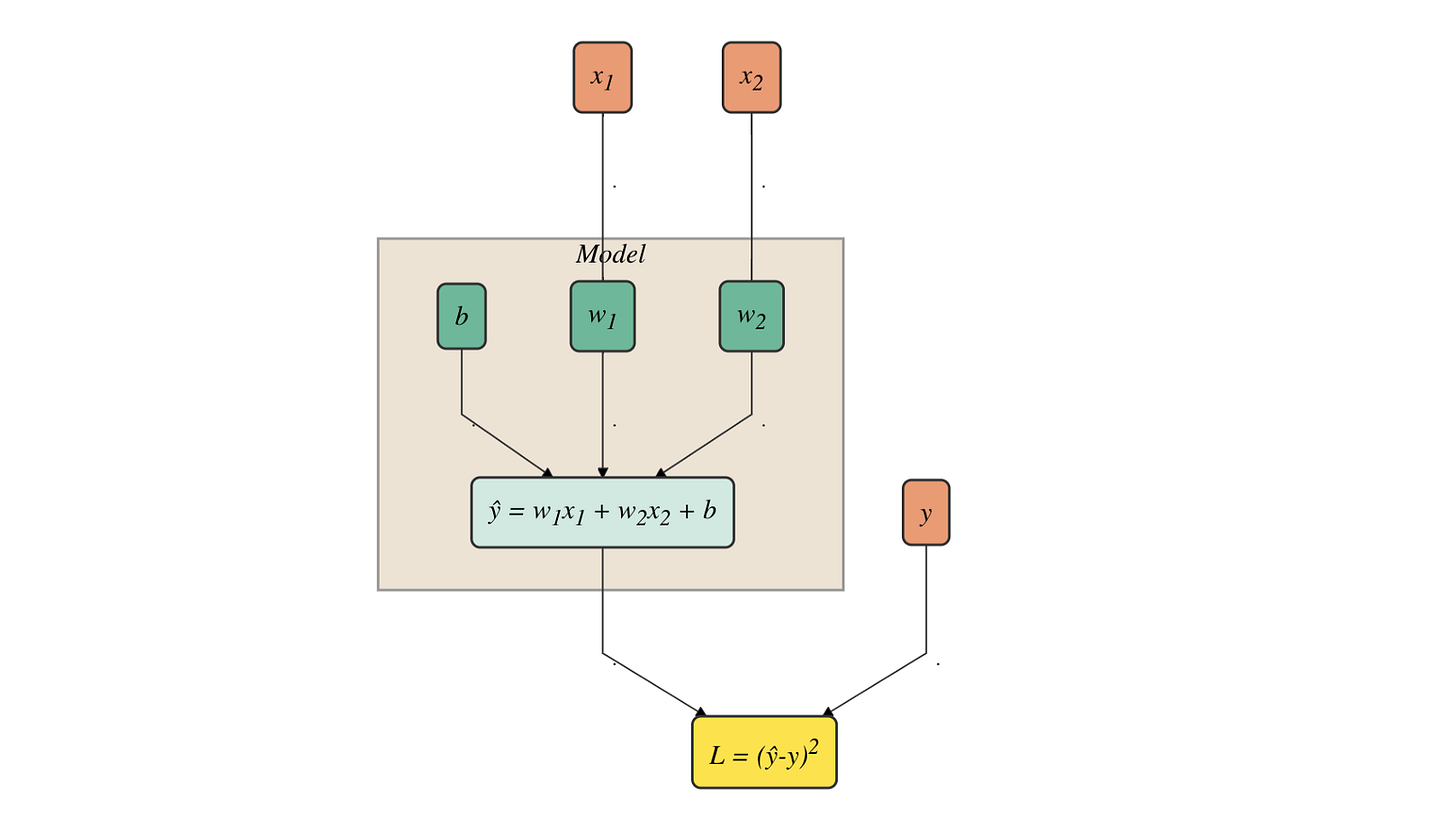Fig. 2.0: Computation graph for linear regression model with stochastic gradient descent.

For forward propagation, you should read this graph from top to bottom and for backpropagation bottom to top.

Note
I have adopted the term ‘placeholder’, a nomenclature used in
TensorFlow to refer to these ‘data variables’.
I will also use the term ‘weights’ to refer to
w and b collectively.

#### 2.1.1 Initialise weights (one-time)

Since gradient descent is all about updating the weights, we need them to start with some values, known as initialising weights.

Here we initialised the weights and bias as follows:

These are reflected in the dark green nodes in Fig. 2.1.1 below: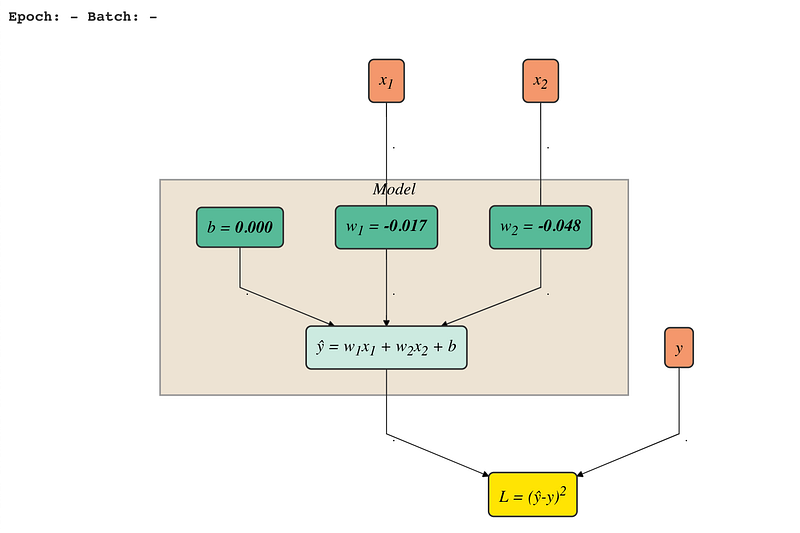Fig. 2.1.1: Weights initialised (dark green nodes)

There are many ways to initialise weights (zeros, ones, uniform distribution, normal distribution, truncated normal distribution, etc.) but we won’t cover them in this post. In this tutorial, we initialised the weights by using truncated normal distribution and the bias with 0.

#### 2.1.2 Feed data

Next, we set the batch size to be 1 and we feed in this first batch of data.

Batch and batch size

We can divide our dataset into smaller groups of equal size. Each group is called a batch and consists of a specified number of examples, called batch size. If we multiply these two numbers, we should get back the number of observations in our data.

Here, our dataset consists of 6 examples and since we defined the batch size to be 1 in this training, we have 6 batches altogether.

Current batch of data used to feed in the model is bolded below:

   x1 x2  y1) 4 1  22) 2 8 -143) 1 0  14) 3 2 -15) 1 4 -76) 6 7 -8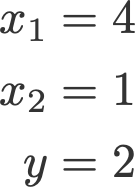Eqn. 2.1.2: First batch of data fed into model

In Fig. 2.1.2, the orange nodes are where we feed in the current batch of data.

Fig. 2.1.2: Feeding data to model with first batch (orange nodes)

#### 2.1.3 Compute ŷ

Now that we have the values of x₁, x₂, w₁, w₂ and b ready, let’s compute ŷ.

The value of ŷ (=-0.1) is reflected in the light green node below: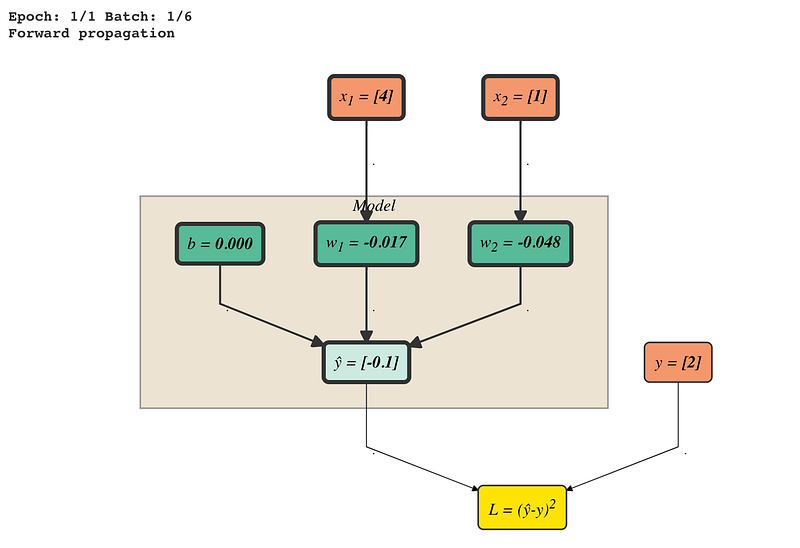Fig. 2.1.3: ŷ computed (light green node)

#### 2.1.4 Compute loss

How far is our predicted ŷ from the given y data? We compare them by calculating the loss function as defined earlier.

You can see this value in the yellow node in the computation graph.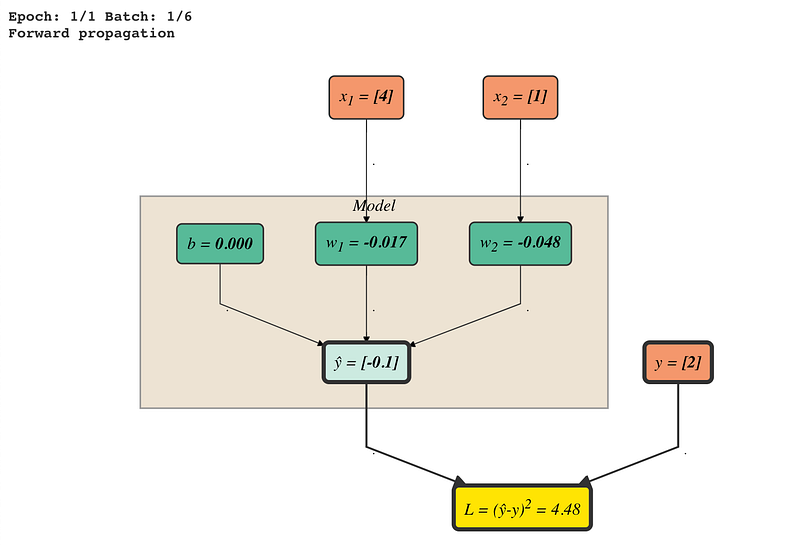Fig. 2.1.4A: L computed (yellow node)

It is a common practise to log the loss during training, together with other information like the epoch, batch and time taken. In my demo, you can see this under the Training progress panel.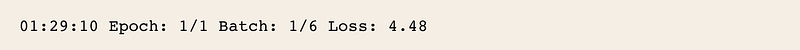Fig. 2.1.4B: Logging loss and other information

#### 2.2.1 Compute partial differentials

Before we start adjusting the values of the weights and bias w₁, w₂ and b, let’s first compute all the partial differentials. These are needed later when we do the weight update.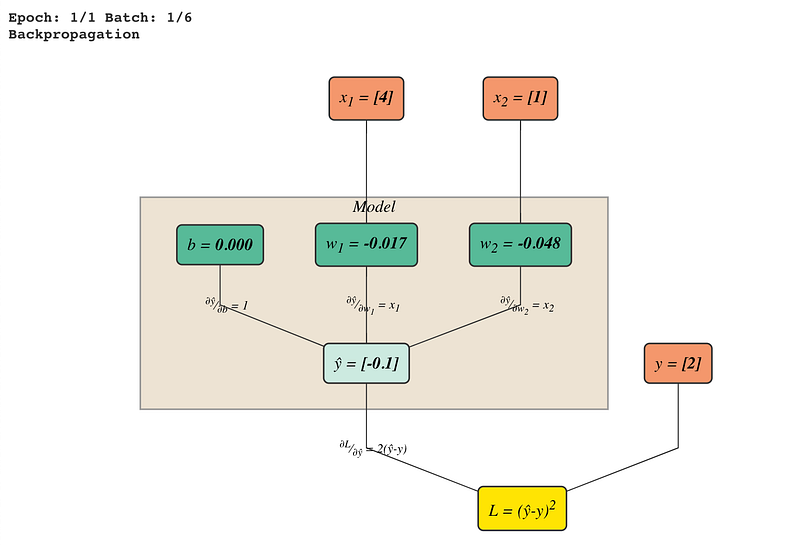Fig. 2.2.1: Indicated partial differentials to the relevant edges on the graph

Namely, we compute all possible paths leading to every w and only, because these are the only variables which we are interested in updating. From Fig. 2.2.1 above, we see that there are 4 edges that we labeled with the partial differentials.

Recall the equations for the model and loss function:

The partial differentials are as follows:

(yellow) — ŷ (light green):

ŷ (light green) — (dark green):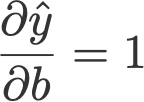Eqn. 2.2.1B: Partial differential of ŷ w.r.t. b

ŷ (light green) — w₁ (dark green):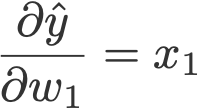Eqn. 2.2.1C: Partial differential of ŷ w.r.t. w1

ŷ (light green) — w₂ (dark green):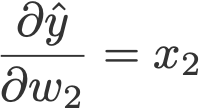Eqn. 2.2.1D: Partial differential of ŷ w.r.t. w2

Note that the values of the partial differentials follow the values from thecurrent batch. For example, in Eqn. 2.2.1C, x₁ = 4.

#### 2.2.2 Update weights

Observe the dark green nodes in Fig. 2.2.2 below. We see three things:
i) b changes from 0.000 to 0.212
ii) w₁ changes from -0.017 to 0.829
iii) w₂ changes from -0.048 to 0.164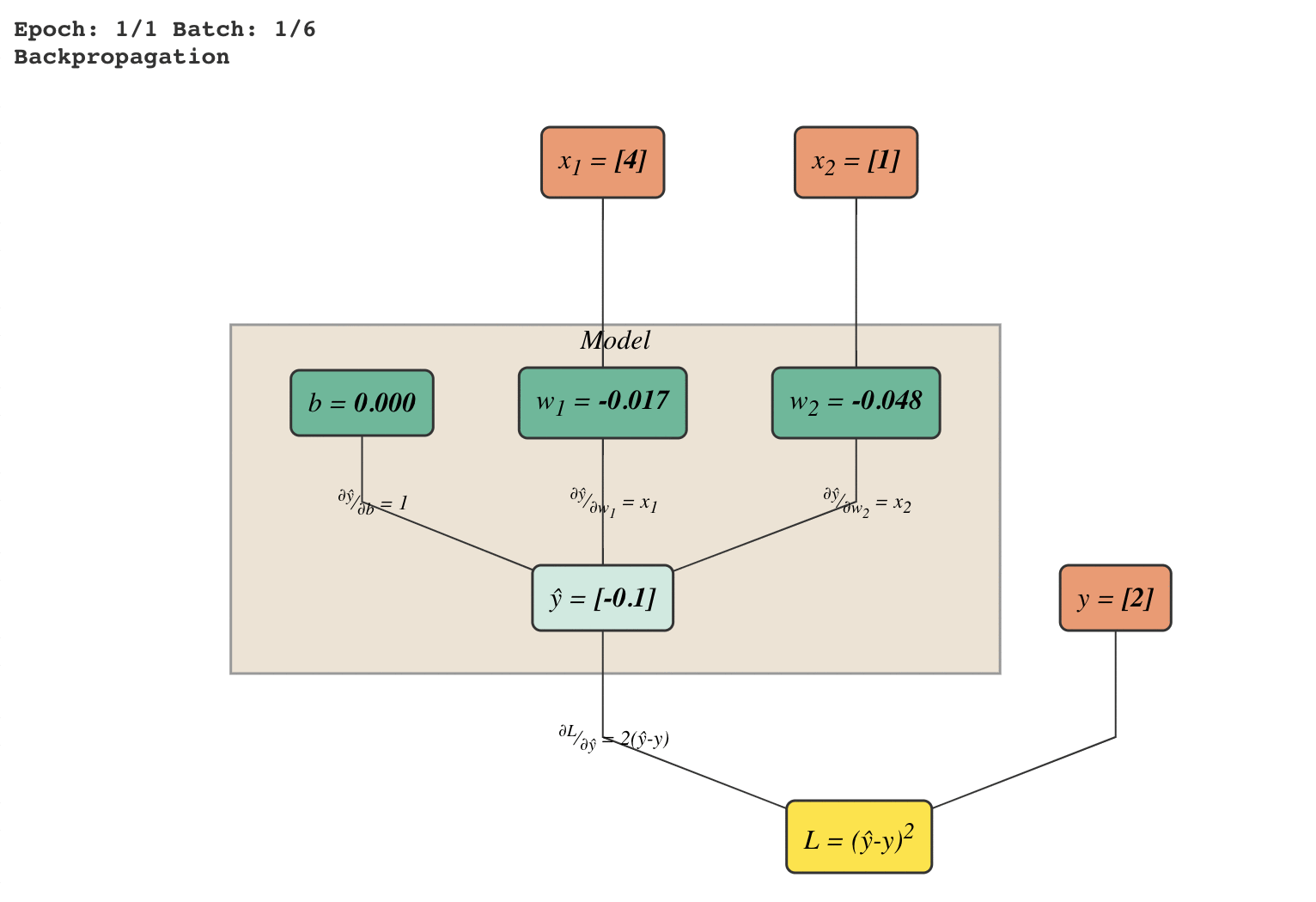Fig. 2.2.2: Updating the weights and bias (dark green nodes)

Also pay attention to the ‘direction’ of the pathway from the yellow node to the green node. They go from bottom to top.

This is stochastic gradient descent — updating the weights using backpropagation, making use of the respective gradient values.

Let’s first focus on updating b. The formula for updating is

where

• b— current value
• b’— value after update
• η —learning rate, set to 0.05
• ∂L/∂b — gradient i.e. partial differential of L w.r.t. b

To get the gradient, we need to multiply the paths from L leading to b using chain rule: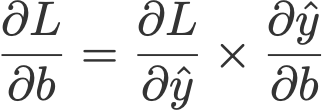Eqn. 2.2.2B: Chain rule for partial differential of L w.r.t. b

We would require the current batch values of xy, ŷ and the partial differentials so let’s just place them below for easy reference: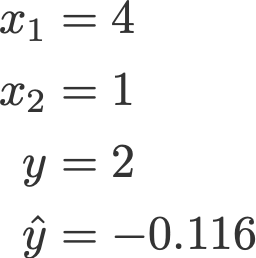Eqn. 2.2.2D: Values from current batch and the predicted ŷ

Using the stochastic gradient descent equation in Eqn. 2.2.2A and plucking in all the values from Eqn. 2.2.2B-D gives us:

That’s it for updating b! Phew! We are left with updating w₁ and w₂, which we update in a similar fashion.

#### End of batch iteration

Congrats! That’s it for dealing with the first batch.

    x1 x2   y
1)   4  1   2  ✔
2)   2  8 -14
3)   1  0   1
4)   3  2  -1
5)   1  4  -7
6)   6  7  -8

Now we need to iterate the above-mentioned steps to the other 5 batches, namely examples 2 to 6.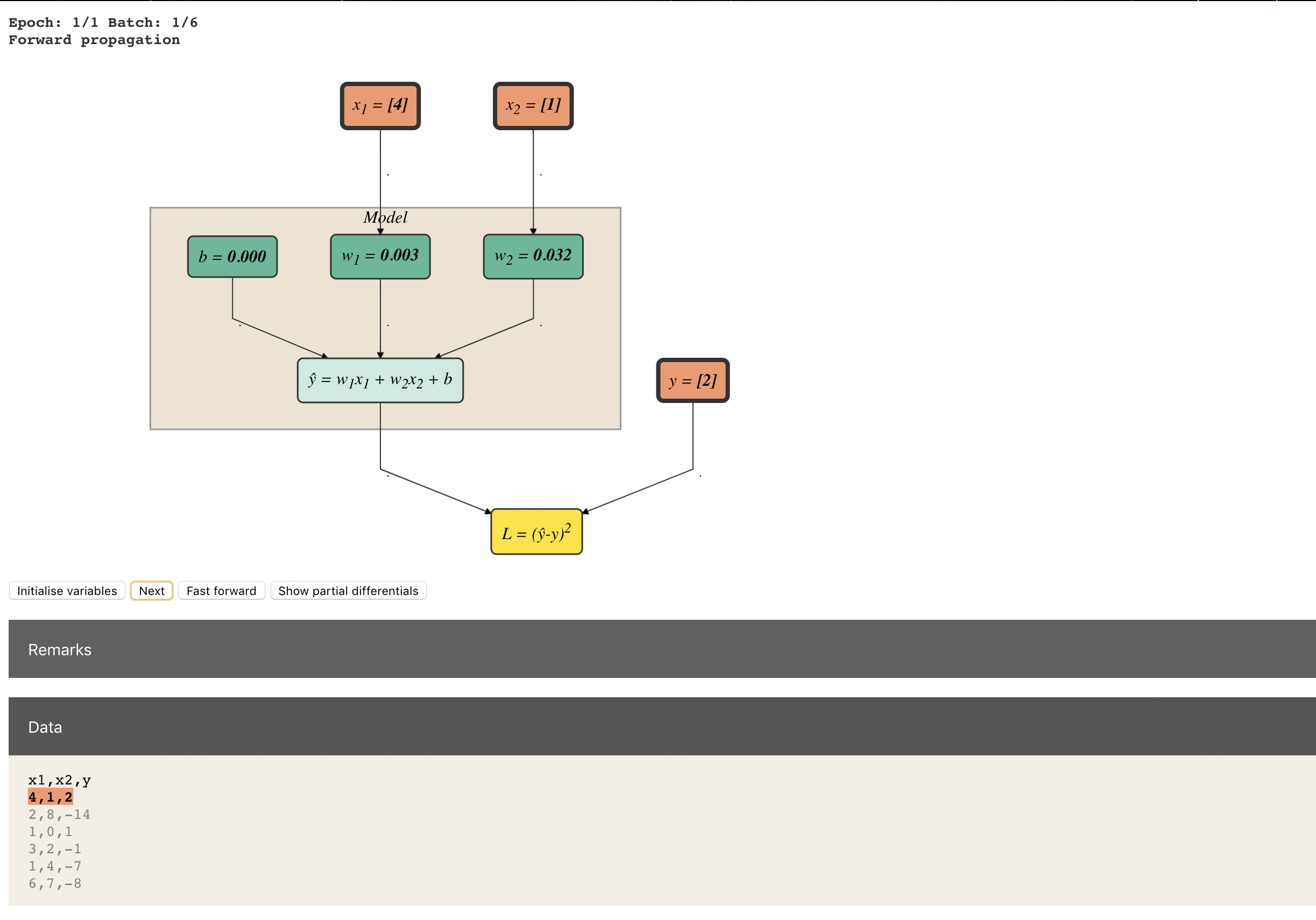Iterating through batch 1 to 6 (apologies for the poor GIF quality! )

#### End of epoch

We complete 1 epoch when the model has iterated through all the batches once. In practise, we extend the epoch to more than 1.

One epoch is when our setup has seen all the observations in our dataset once. But one epoch is almost always never enough for the loss to converge. In practice, this number is manually tuned.

At the end of it all, you should get a final model, ready for inference, say:

Let’s have a review of the entire workflow in a pseudo-code:

initialise_weights()

for i in epochs:

for j in batches:

#forward propagation
feed_batch_data()
compute_ŷ()
compute_loss()

#backpropagation
compute_partial_differentials()
update_weights()

#### Improve training

One epoch is never enough for a stochastic gradient descent optimisation problems. Remember that in Fig. 4.1, our loss is at 4.48. If we increase the number of epochs, which means just increasing the number of times we update the weights and biases, we can converge it to a satisfactory low.

Below are the things you can improve the training:

• Extend training to more than 1 epoch
• Increase batch size
• Change optimiser (see my post on gradient descent optimisation algorithms here)
• Adjust learning rate (changing the learning rate value or using learning rate schedulers)
• Hold out a train-val-test set

I built an interactive explorable demo on linear regression with gradient descent in JavaScript. Here are the libraries I used:

• Dagre-D3 (GraphViz + d3.js) for rendering the graphs
• MathJax for rendering mathematical notations
• ApexCharts for plotting line charts
• jQuery

Check out the interactive demo here.

You might also like to check out A Line-by-Line Layman’s Guide to Linear Regression using TensorFlow, which focuses on coding linear regression using the TensorFlow library.

#### References

https://colah.github.io/posts/2015-08-Backprop/

#### Related Articles on Deep Learning

Animated RNN, LSTM and GRU

Line-by-Line Word2Vec Implementation (on word embeddings)

10 Gradient Descent Optimisation Algorithms + Cheat Sheet

Counting No. of Parameters in Deep Learning Models

Attn: Illustrated Attention

Thanks to Ren Jie and Derek for ideas, suggestions and corrections to this article.

Follow me on Twitter @remykarem for digested articles and demos on AI and Deep Learning.

## Intuitions on L1 and L2 Regularisation

This article was first published in Towards Data Science. Photo by rawpixel on Unsplash.

Overfitting is a phenomenon that occurs when a machine learning or statistics model is tailored to a particular dataset and is unable to generalise to other datasets. This usually happens in complex models, like deep neural networks.

Regularisation is a process of introducing additional information in order to prevent overfitting. The focus for this article is L1 and L2 regularisation.

There are many explanations out there but honestly, they are a little too abstract, and I’d probably forget them and end up visiting these pages, only to forget again. In this article, I will be sharing with you some intuitions why L1 and L2 work using gradient descent. Gradient descent is simply a method to find the ‘right’ coefficients through (iterative) updates using the value of the gradient. (This article shows how gradient descent can be used in a simple linear regression.)

Let’s go!

### 0) L1 and L2

L1 and L2 regularisation owes its name to L1 and L2 norm of a vector respectively. Here’s a primer on norms: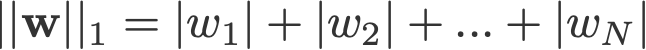1-norm (also known as L1 norm)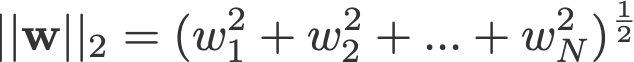2-norm (also known as L2 norm or Euclidean norm)

A linear regression model that implements L1 norm for regularisation is called lasso regression, and one that implements L2 norm for regularisation is called ridge regression. To implement these two, note that the linear regression model stays the same:

but it is the calculation of the loss function that includes these regularisation terms:

Note: Strictly speaking, the last equation (ridge regression) is a loss function with squared L2 norm of the weights (notice the absence of the square root). (Thank you Max Pechyonkin for highlighting this!)

The regularisation terms are ‘constraints’ by which an optimisation algorithm must ‘adhere to’ when minimising the loss function, apart from having to minimise the error between the true y and the predicted ŷ.

### 1) Model

Let’s define a model to see how L1 and L2 work. For simplicity, we define a simple linear regression model ŷ with one independent variable.

Here I have used the deep learning conventions w (‘weight’) and (‘bias’).

In practice, simple linear regression models are not prone to overfitting. As mentioned in the introduction, deep learning models are more susceptible to such problems due to their model complexity.

As such, do note that the expressions used in this article are easily extended to more complex models, not limited to linear regression.

### 2) Loss Functions

To demonstrate the effect of L1 and L2 regularisation, let’s define 3 loss functions:

• L
• L1
• L2

Our objective is to minimise these different losses.

#### 2.1) Loss function with no regularisation

We define the loss function L as the squared error, where error is the difference between y (the true value) and ŷ (the predicted value).

Let’s assume our model will be overfitted using this loss function.

#### 2.2) Loss function with L1 regularisation

Based on the above loss function, adding an L1 regularisation term to it looks like this:

where the regularisation parameter λ > 0 is manually tuned. Let’s call this loss function L1. Note that |w| is differentiable everywhere except when w=0, as shown below. We will need this later.

#### 2.3) Loss function with L2 regularisation

Similarly, adding an L2 regularisation term to L looks like this:

where again, λ > 0.

### 3) Gradient descent

Now, let’s solve the linear regression model using gradient descent optimisation based on the 3 loss functions defined aboveRecall that updating the parameter in gradient descent is as follows:

Let’s substitute the last term in the above equation with the gradient of LL1 and L2 w.r.t. w.

L:

L1:

L2:

### 4) How is overfitting prevented?

From here onwards, let’s perform the following substitutions on the equations above (for better readability):

• η = 1,
• H = 2x(wx+b-y)

which give us

L:

L1:

L2:

#### 4.1) With vs. Without Regularisation

Observe the differences between the weight updates with the regularisation parameter λ and without it.

Intuition A:

Let’s say with Equation 0, calculating w-H gives us a value that leads to overfitting. Then, intuitively, Equations {1.1, 1.2 and 2} will reduce the chances of overfitting because introducing λ makes us shift away from the very w that was going to cause us overfitting problems in the previous sentence.

Intuition B:

Let’s say an overfitted model means that we have a w value that is perfect for our model. ‘Perfect’ meaning if we substituted the data (x) back in the model, our prediction ŷ will be very, very close to the true y. Sure, it’s good, but we don’t want perfect. Why? Because this means our model is only meant for the dataset which we trained on. This means our model will produce predictions that are far off from the true value for other datasets. So we settle for less than perfect, with the hope that our model can also get close predictions with other data. To do this, we ‘taint’ this perfect w in Equation 0 with a penalty term λ. This gives us Equations {1.1, 1.2 and 2}.

Intuition C:

Notice that (as defined here) is dependent on the model (w and b) and the data (and y). Updating the weights based only on the model and data in Equation 0 can lead to overfitting, which leads to poor generalisation. On the other hand, in Equations {1.1, 1.2 and 2}, the final value of w is not only influenced by the model and data, but also by a predefined parameter λ which is independent of the model and data. Thus, we can prevent overfitting if we set an appropriate value of λ, though too large a value will cause the model to be severely underfitted.

#### 4.2) L1 vs. L2

We shall now focus our attention to L1 and L2, and rewrite Equations {1.1, 1.2 and 2} by rearranging their λ and H terms as follows:

L1:

L2:

Compare the second term of each of the equation above. Apart from H, the change in w depends on the ±λ term or the -2λw term, which highlight the influence of the following:

1. sign of current w (L1, L2)
2. magnitude of current w (L2)
3. doubling of the regularisation parameter (L2)

While weight updates using L1 are influenced by the first point, weight updates from L2 are influenced by all aspects. While I have made this comparison just based on the iterative equation update, please note that this does not mean that one is ‘better’ than the other.

For now, let’s see below how a regularisation effect from L1 can be attained just by the sign of the current w.

#### 4.3) L1’s effect on pushing towards 0 (sparsity)

Take a look at L1 in Equation 3.1. If w is positive, the regularisation parameter λ>0 will push w to be less positive, by subtracting λ from w. Conversely in Equation 3.2, if w is negative, λ will be added to w, pushing it to be less negative. Hence, this has the effect of pushing w towards 0.

This is of course pointless in a 1-variable linear regression model, but will prove its prowess to ‘remove’ useless variables in multivariate regression models. You can also think of L1 as reducing the number of features in the model altogether. Here is an arbitrary example of L1 trying to ‘push’ some variables in a multivariate linear regression model:

So how does pushing w towards 0 help in overfitting in L1 regularisation? As mentioned above, as goes to 0, we are reducing the number of features by reducing the variable importance. In the equation above, we see that x_2x_4 and x_5 are almost ‘useless’ because of their small coefficients, hence we can remove them from the equation. This in turn reduces the model complexity, making our model simpler. A simpler model can reduce the chances of overfitting.

#### Note

While L1 has the influence of pushing weights towards 0 and L2 does not, this does not imply that weights are not able to reach close to 0 due to L2.

### References

Norm (Mathematics) (wikipedia.org)

Lasso (Statistics) (wikipedia.org)

Lasso and Ridge Regularization (medium.com)

Special thanks to Yu Xuan, Ren Jie, Daniel and Derek for ideas, suggestions and corrections to this article. Also thank you C Gam for pointing out the mistake in the derivative.

Follow me on Twitter @remykarem or LinkedIn. You may also reach out to me via raimi.bkarim@gmail.com. Feel free to visit my website at remykarem.github.io.

## Developing Local Talent Key for Singapore in AI Race (Source: Straits Times, 29 March 2019)

“Immediate need for locals to plug AI talent gap in S’pore,” said retired Israeli major-general Isaac Ben-Israel.

In this article, Prof Ben-Israel commented that Singapore has the advantage of being small and nimble, with a leadership that can move things “very quickly”. He added that while we can temporarily fill the talent gap by using foreigners, we must develop our own people to meet national demands over the long term. And that development is already underway – he referenced 2 of AI Singapore’s programmes to equip and enable AI knowledge and talent i.e. AI for Industry and AI for Everyone.

Prof Ben-Israel also visited AI Singapore and mingled with some of our Batch 2 apprentices during the tour.

## A look at its history, potential, progress, and challenges

A few weeks ago, I attended the industry workshop Translating AI Technology into Healthcare Solutions organized by AI Singapore (pictured below). Among the many interesting topics discussed was the decentralized collaborative machine learning approach known as federated learning. It piqued my interest and I decided to read more about it.Workshop panelists from left to right : Dr Stefan Winkler (moderator), Deputy Director, AI Singapore; Trent McConaghy, Founder, Ocean Protocol; Dr Ronald Ling, CEO, Connected Health; Lance Little, Managing Director for Asia Pacific, Roche Diagnostics; Dan Vahdat, Co-founder & CEO, Medopad; Dr Joydeep Sarkar, Chief Analytics Officer, Holmusk; Dr Yu Han, Assistant Professor, Nanyang Technological University; Dr Khung Keong Yeo, Senior Consultant of the Dept of Cardiology, National Heart Centre Singapore

### History

The term Federated Learning was coined by Google in a paper first published in 2016. Since then, it has been an area of active research as evidenced by papers published on arXiv. In the recent TensorFlow Dev Summit, Google unveiled TensorFlow Federated (TFF), making it more accessible to users of its popular deep learning framework. Meanwhile, for PyTorch users, the open-source community OpenMined had already made available the PySyft library since the end of last year with a similar goal (linklink).

### What is Federated Learning?

Federated learning is a response to the question: can a model be trained without the need to move and store the training data to a central location?

It is a logical next step in the ongoing integration of machine learning into our daily lives, prompted by existing constraints and also other concurrent developments.

Islands of Data

Much of the world’s data today sit, not on central data centers, but on isolated islands where they are collected and owned. In other words, much potential could be tapped if they could be worked upon where they sit.

Data Privacy

The issue of data privacy has come under the increasing attention of regulators in several jurisdictions in recent years (link). With data availability essential to any machine learning model, creative ways must be devised to circumvent restrictions and enable model training to happen without the data actually having to leave where it is collected and stored.

Computing on the Edge

As will be explained later, federated learning often requires computing on the edge. For edge devices (primarily handsets) that collect and store data, recent advances in custom hardware (for example, Apple’s Neural Engine) have made deep learning on them feasible. This has been the case since the introduction of the Samsung S9 and Apple X series of handsets. As the number of these so-called “AI-ready” handsets in the market grows (link), the potential for federated learning does too.

### What Federated Learning Promises

Federated learning also has the potential to act as the impetus to future changes in the industry.

Cloud Computing

Cloud computing is the dominant computing paradigm for machine learning today in a space occupied by the tech giants Google, Amazon, and Microsoft. Without the need to maintain a central data center, new providers will find it easier to offer even more AI services. The fact is, Google has foreseen this democratizing trend and has staked a leading role in the development of federated learning.

Sharing Economy

Google used federated learning to develop its next-word predictor on GBoard. This ability to train a model without compromising users’ privacy should encourage the emergence of other services that rely on data collected through handsets and other IoT devices in a form of sharing economy.

B2B Collaboration

Since data never leaves its original premises, federated learning opens up the possibility for different data owners at the organizational level to collaborate and share their data. In a recent paper, the researchers (Qiang Yang et al.) envision the different configurations in which this can happen.

They coined the terms Horizontal Federated Learningand Vertical Federated Learning.

Take the case of two regional banks. Although they have non-overlapping clientele, their data will have similar feature spaces since they have very similar business models. They might come together to collaborate in an example of horizontal federated learning.

In vertical federated learning, two companies providing different services (e.g. banking and e-commerce) but having a large intersection of clientele might find room to collaborate on the different feature spaces they own, leading to better outcomes for both.

In both cases, the data owners are able to collaborate without having to sacrifice their respective clientele’s privacy.

Apart from finance, another industry vertical that could benefit is the healthcare sector (as mentioned in the introduction). Hospitals and other healthcare providers stand to gain if they are able to share patient data for model training in a privacy-preserving manner.

### How Federated Learning Works

At the heart of federated learning is the federated averaging algorithm introduced by Google in their original paper (pseudo-code shown below).

A typical round of learning consists of the following sequence.

• A random subset of members of the Federation (known as clients) is selected to receive the global model synchronously from the server.
• Each selected client computes an updated model using its local data.
• The model updates are sent from the selected clients to the server.
• The server aggregates these models (typically by averaging) to construct an improved global model.

Of course, the subset selection step was necessitated by the context in which Google originally applied federated learning: on data collected through millions of handsets in its Android ecosystem.

A variant of this learning sequence which appears in later literature involves sending gradient updates to the server instead of the actual model weights themselves. The common idea is that none of the original data is ever transmitted between parties, only model-related updates. It is also clear now how edge computing plays a role here.

### Challenges of Federated Learning

Moving federated learning from concept to deployment is not without challenges. Researchers, including those working independently of federated learning advocates, have contributed to a better understanding of the issues to be considered. While work has been done on the efficiency and accuracy of federated learning, the more important challenges, in my opinion, are the security-related ones mentioned below.

Inference Attacks (linklinklinklinklink). The motivation for federated learning is the preservation of the privacy of the data owned by the clients. Even when the actual data is not exposed, the repeated model weight updates can be exploited to reveal properties not global to the data but specific to individual contributors. This inference can be performed on both the server side as well as (other) client side. An oft-quoted countermeasure is the use of differential privacy to mitigate this risk.

Model Poisoning (linklinklink). Some researchers have investigated the possibility of misbehaving clients introducing backdoor functionality or mounting Sybil attacks to poison the global model.Targeted poisoning attack in stochastic gradient descent. The dotted red vectors are Sybil contributions that drive the model towards a poisoner objective. The solid green vectors are contributed by honest clients that drive towards the true objective.

To effectively counter such attacks, additional overhead for Sybil detection must be considered.

Federated learning holds the promise to make available more data to improve lives. Much research is being undertaken and many challenges remain. Perhaps the following paragraph from Google’s latest paper sums up today’s status.

In this paper, we report on a system design for such algorithms in the domain of mobile phones (Android). This work is still in an early stage, and we do not have all problems solved, nor are we able to give a comprehensive discussion of all required components. Rather, we attempt to sketch the major components of the system, describe the challenges, and identify the open issues, in the hope that this will be useful to spark further systems research.

The original article first appeared here.

## Who is responsible? The ethics and societal implications of AI

Professor Leong Tze Yun, Director of AI Technology, provided her insights on the power and limitations of AI during a panel discussion at the recent Times Higher Education Innovation and Impact Summit that was held in South Korea.

Click here to view the full video.